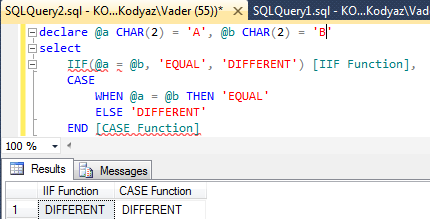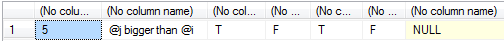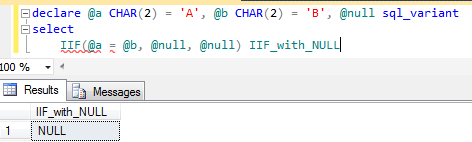SQL Server 2012 Download and T-SQL Tutorials Development resources, articles, tutorials, code samples and tools and downloads for ASP.Net, SQL Server, R Script, Windows, Windows Phone, AWS, SAP HANA and ABAP, like SAP UI5, Screen Personas, etc.
 Home Articles News IT Jobs Tools Sample Chapters Trainers Blogs Forums Photos Files

SQL Server 2019 Installation

# New SQL IIF Boolean Function in Transact-SQL with SQL Server 2012

SQL IIF function evaluates a boolean expression and according to the being True or False of the boolean expression, returns one of the input values. Application developers and programmers are familiar with IIF() function since many programming languages have IIF() boolean functions.

T-SQL IIF() boolean function is introduced to sql developes with SQL Server 2012, Denali CTP3. This new SQL IIF() function syntax is as follows:

IIF([boolean expression], [true value], [false value])

For T-SQL developers, IIF() function can be considered as a special type of SQL CASE() function. Let's make a sample SQL script to see how sql programmers can use iif() function instead of Case statement.

``` declare @a CHAR(1) = 'A', @b CHAR(1) = 'B' select  IIF(@a = @b, 'EQUAL', 'DIFFERENT') [IIF Function],  CASE   WHEN @a = @b THEN 'EQUAL'   ELSE 'DIFFERENT'  END [CASE Function] ```

As you have expected the output of the above two functions are the sameSQL IIF() function has some additional behaviours that tsql developers should consider while coding especially related with NULL input parameters. Although the below SELECT statements returns valid values from SQL IIF() functions,

``` declare @i int = 3, @j int = 5 declare @f bit = 1 declare @null int select  IIF(@i > @j, @i, @j),  IIF(@i > @j, '@i bigger than @j', iif(@j > @i, '@j bigger than @i', '@i equal to @j')),  IIF(1=1, 'T','F'),  IIF(@null = @null, 'T','F'),  IIF(NULL IS NULL, 'T','F'),  IIF(NULL = NULL, 'T','F'),  IIF(2 > 1, NULL, 'F') ```The following IIF() function returns error message. Since the input values for TRUE and FALSE result of the boolean expression are both NULL, the SQL Engine throws the error message 8133.

``` select IIF(2 > 1, NULL, NULL) ```

Msg 8133, Level 16, State 1, Line 2
At lease one of the result expressions in a CASE specification must be an expression other than the NULL constant.

But if both TRUE and FALSE evaluation of the boolean expression resulting into variables which have NULL values, then the SQL IIF() function call executes successfully

``` declare @a CHAR(2) = 'A', @b CHAR(2) = 'B', @null sql_variant select IIF(@a = @b, @null, @null) ```Related SQL Resources

SQL Server Articles

SQL Server 2012

SQL Server Tools

SQL Blog

MS SQL Server Forums